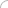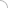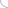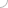Logical Reasoning## Online logical reasoning test - Part 2

11. Put + or – between the numbers to obtain the given result. 40 10 10 60 2 = 98

a.) + + - +
b.) + + - -
c.) - + + -
d.) + - + +

ANSWER : - + + -

C, F, I, L, O, ?

a.) P
b.) Q
c.) R
d.) S
e.) T

##### 13. In the given series, which alphabet would come in place of ‘?’
T, V, Z, B, F, ‘?’

a.) G
b.) H
c.) K
d.) J
e.) I

##### 14. In the given series, which alphabet would come in place of ‘?’ Y, W, U, S, Q, ‘?’

a.) A
b.) P
c.) O
d.) B
e.) M

15. In a certain language CONSTABLE is coded as 91, what will be the code for STABLE?

a.) 97
b.) 59
c.) 79
d.) 75
e.) 67

a.) 2
b.) 1
c.) 3
d.) 4
e.) 7

a.) ESPBN
b.) ETIEQ
c.) ETHER
d.) ESHDR

a.) QAMMSPB
b.) QAMTRBP
c.) STAAUSRP
d.) QAMMRCP

##### 19. In a certain language BODY is written as APCZ, how will DELHI be written in that language?

a.) CFKIH
b.) CFMIJ
c.) BFKGI
d.) BFKIHAptitude questions